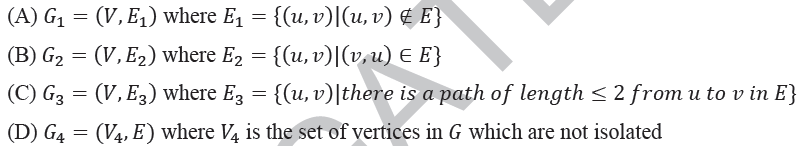# GATE | GATE-CS-2014-(Set-1) | Question 13

Let G=(V,E) be a directed graph where V is the set of vertices and E the set of edges. Then which one of the following graphs has the same strongly connected components as G ?(A) A
(B) B
(C) C
(D) D